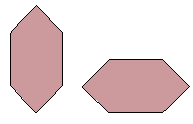Name: ___________________Date:___________________

 Email us to get an instant 20% discount on highly effective K-12 Math & English kwizNET Programs!

### Grade 4 - Mathematics12.4 Similar and Congruent Figures

 Two figures are similar if they are of the same shape, not necessarily the size. Two figures are said to be congruent, if they are of the same shape and size. Congruent figures can be similar but the vice versa is always not true. Example: The two figures areAnswer: similar and not congruent Directions: Check the following figures foe congruence and similarity.
 Q 1: The two figures arenot similarnot congruent Q 2: The two figures are2similar and congruentsimilar and not congruent Q 3: The two figures arecongruentsimilar Q 4: The two figures arenot similarnot congruent Question 5: This question is available to subscribers only! Question 6: This question is available to subscribers only!